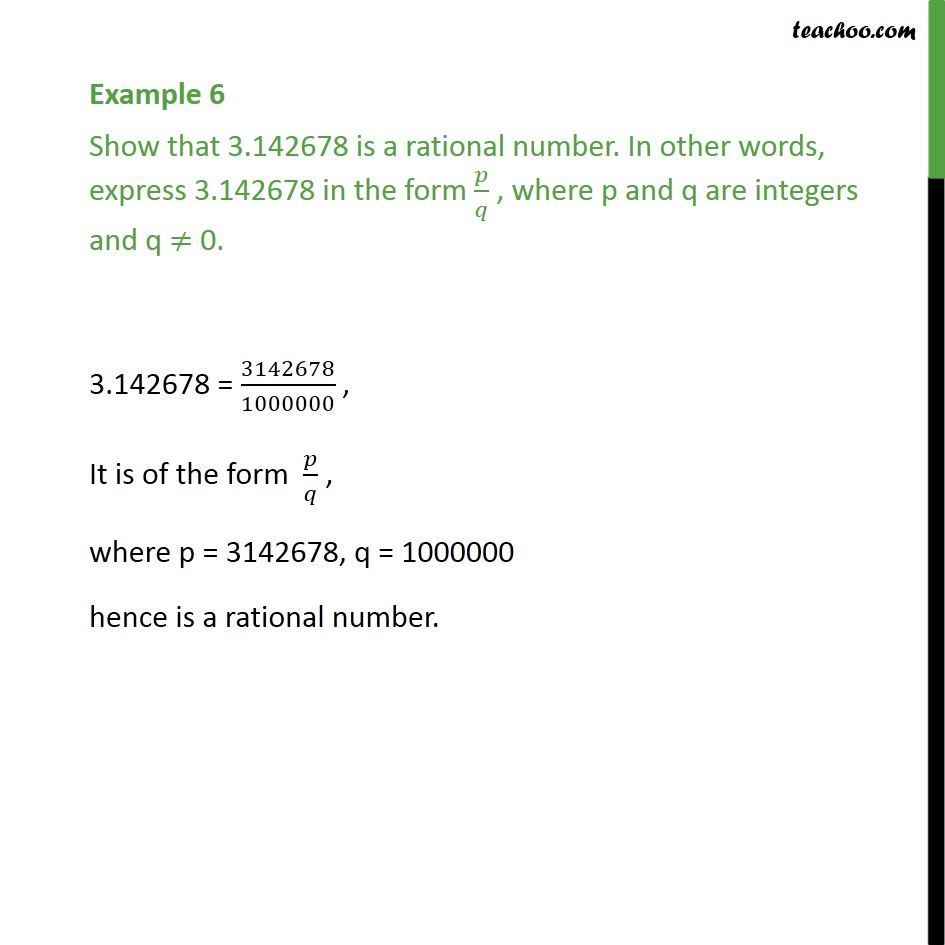1. Chapter 1 Class 9 Number Systems (Term 1)
2. Concept wise
3. Expressing decimal in p/q

Transcript

Example 6 Show that 3.142678 is a rational number. In other words, express 3.142678 in the form 𝑝/𝑞 , where p and q are integers and q ≠ 0. 3.142678 = 3142678/1000000 , It is of the form 𝑝/𝑞 , where p = 3142678, q = 1000000 hence is a rational number.

Expressing decimal in p/q